# RS Aggarwal Solutions for Class 9 Maths Chapter 13: Geometrical Constructions

Geometrical Constructions are very important as it is used in many fields. Therefore, it is important for the students to learn the concepts and understand their uses in day to day life. The best way to understand the concepts is by downloading the PDF of solutions prepared by our expert team of faculties. The concepts are articulated by experts in an efficient manner in order to improve the exam performance of the students.

RS Aggarwal Solutions provides answers in a comprehensive way to help students understand the concepts in a better way. The explanations for each problem provided by the faculties are clear cut and simple. RS Aggarwal Solutions for Class 9 Chapter 13 Geometrical Constructions are provided here.

## RS Aggarwal Solutions for Class 9 Maths Chapter 13: Geometrical Constructions Download PDF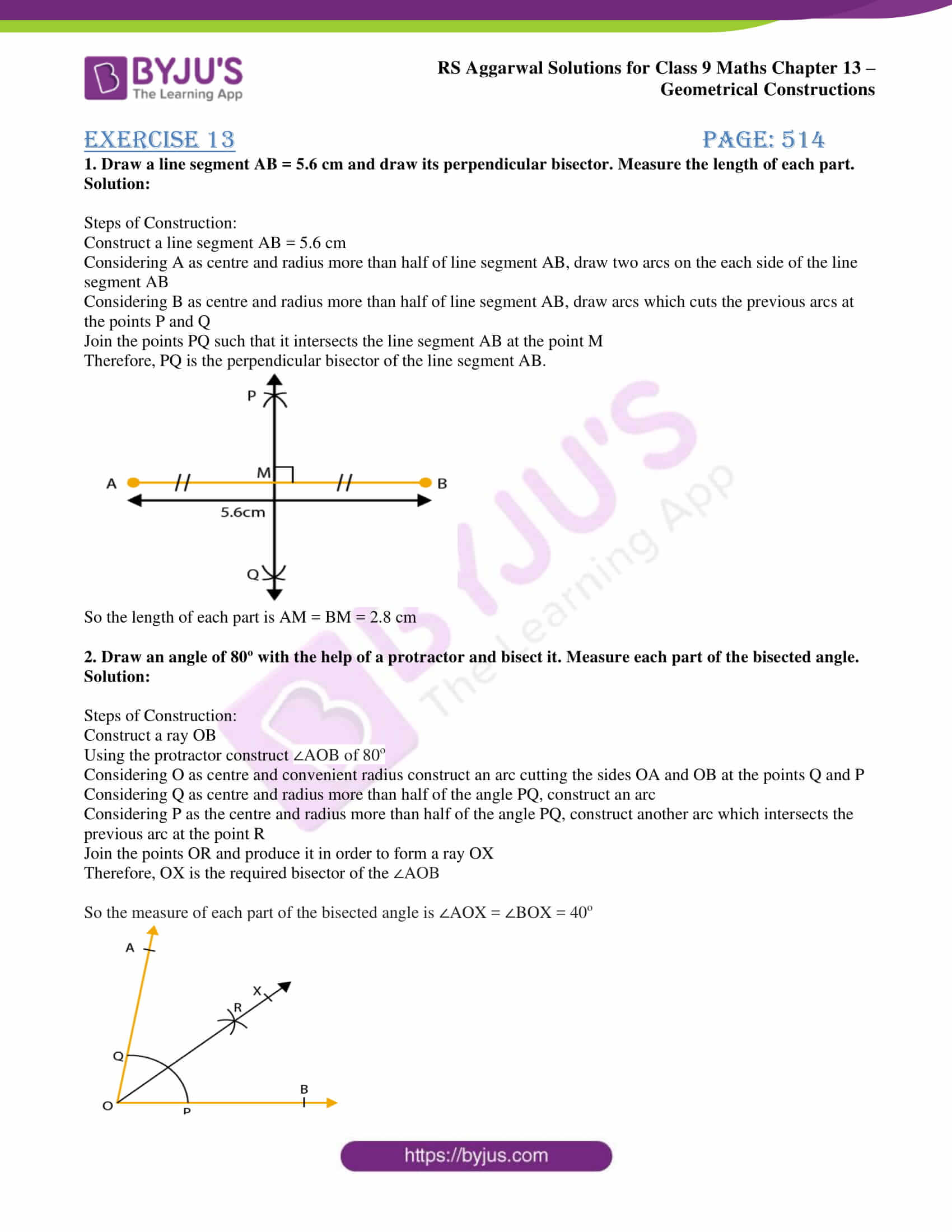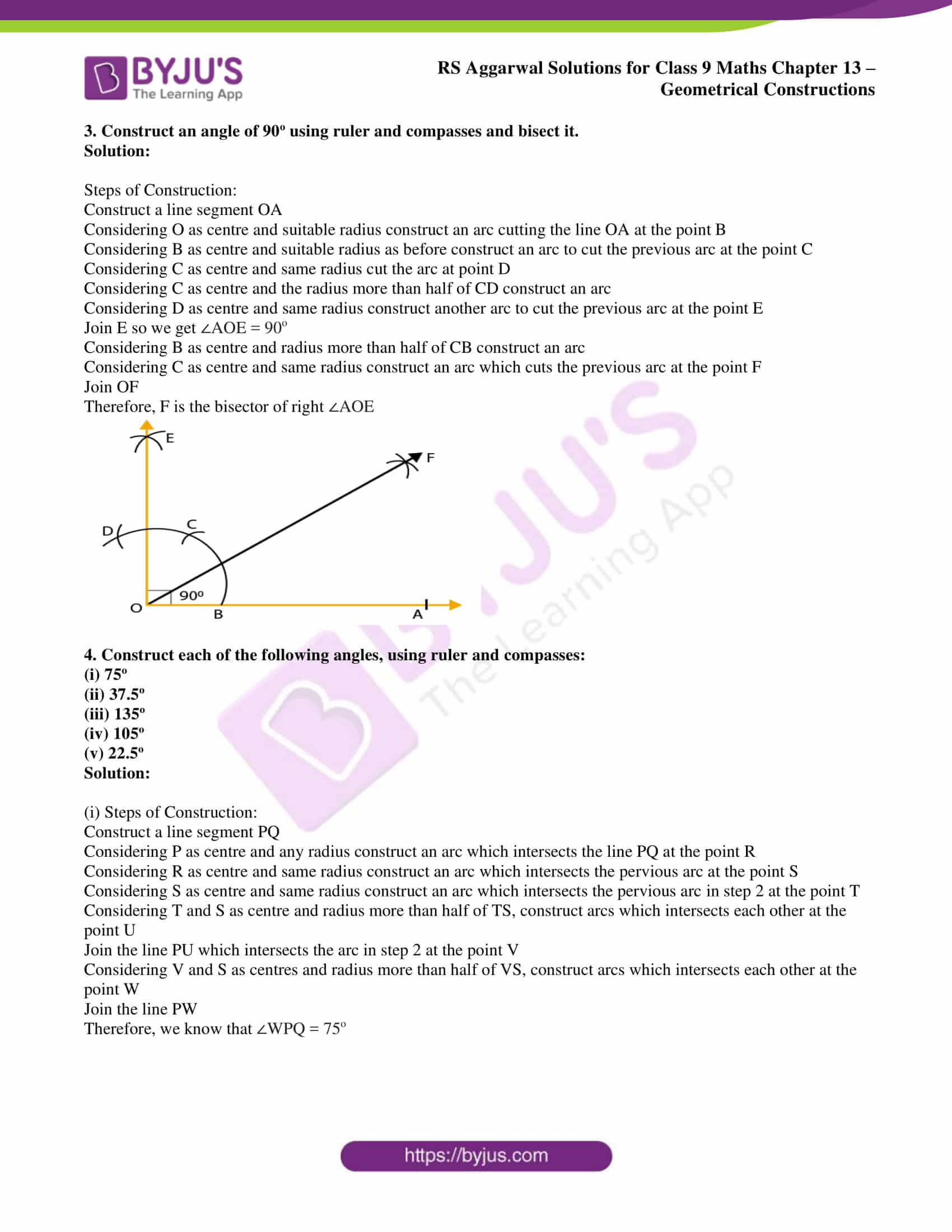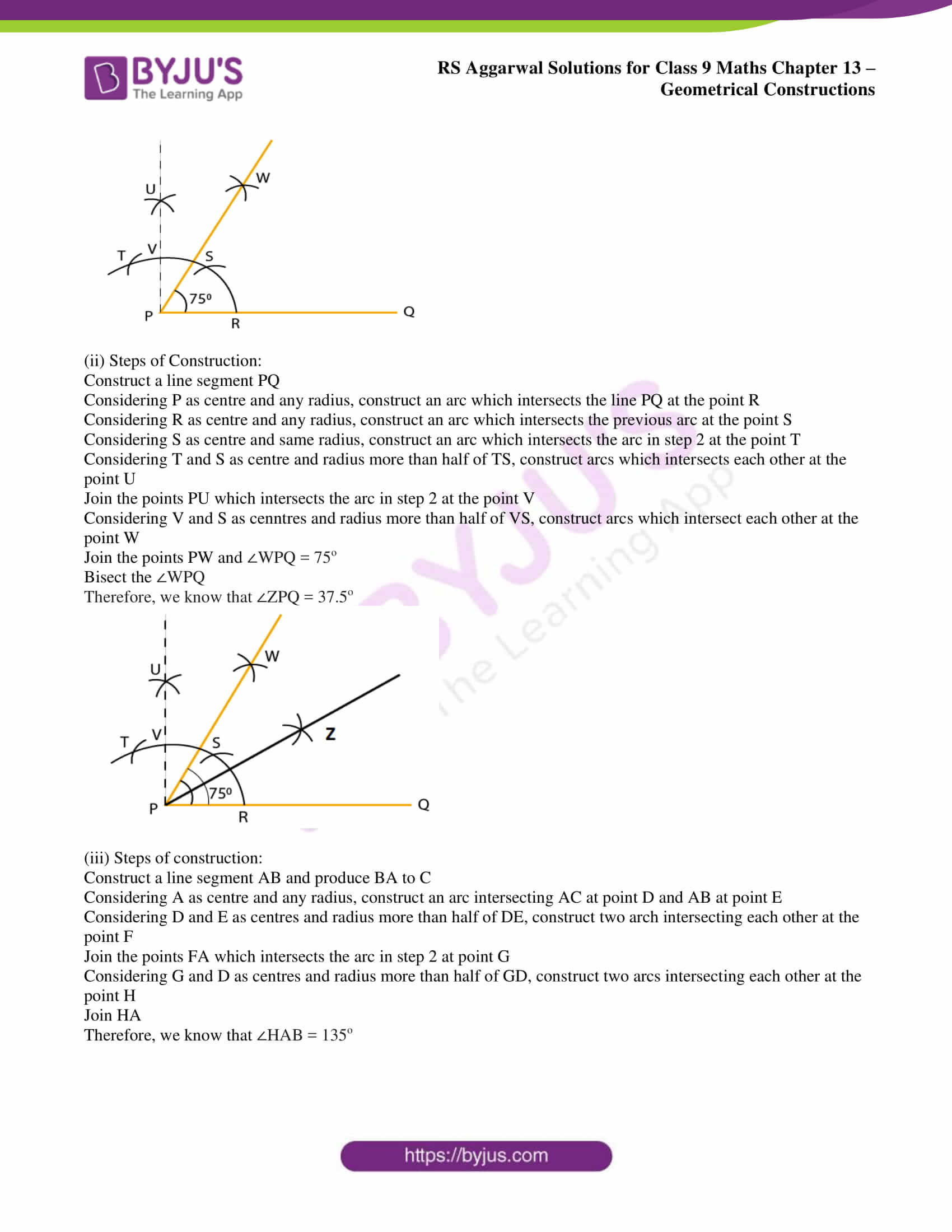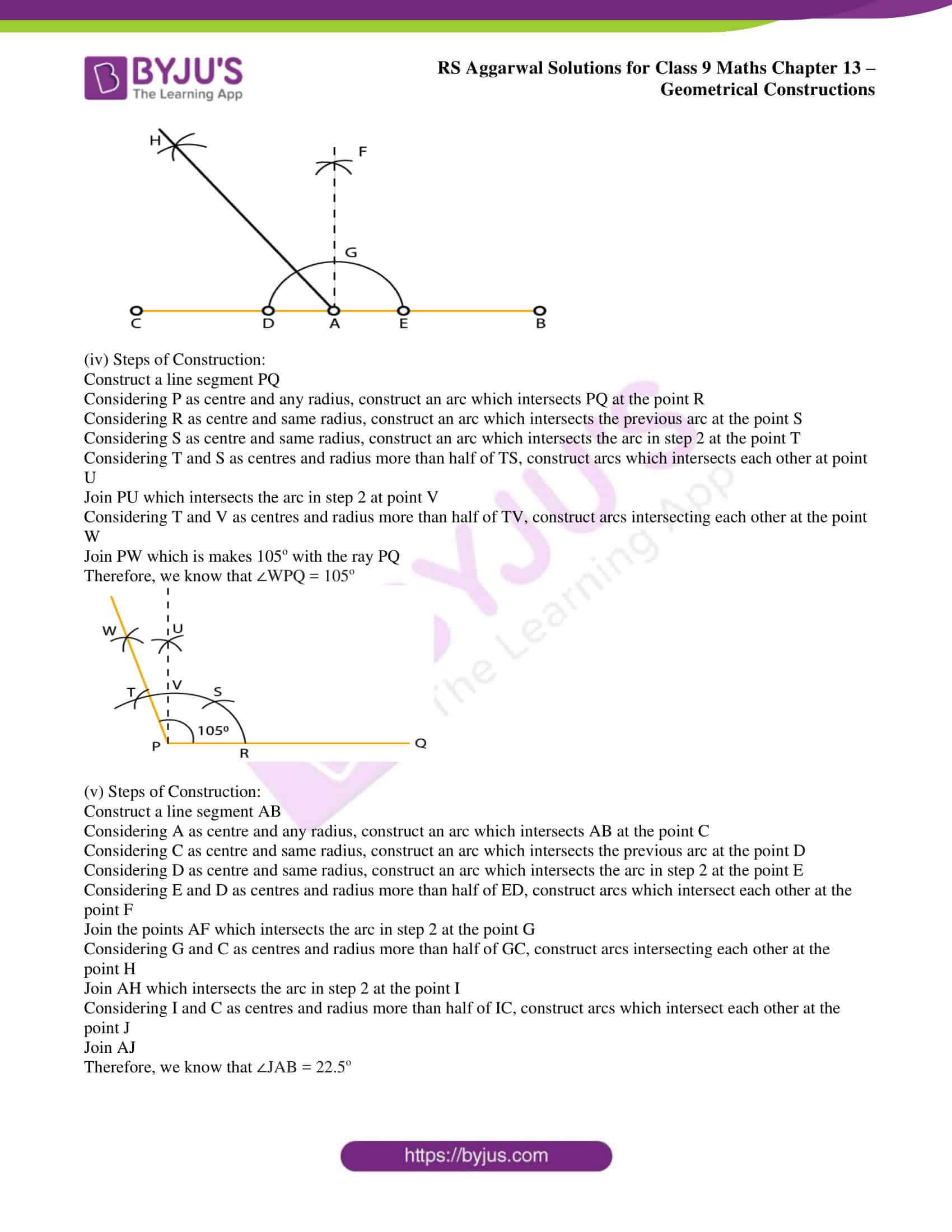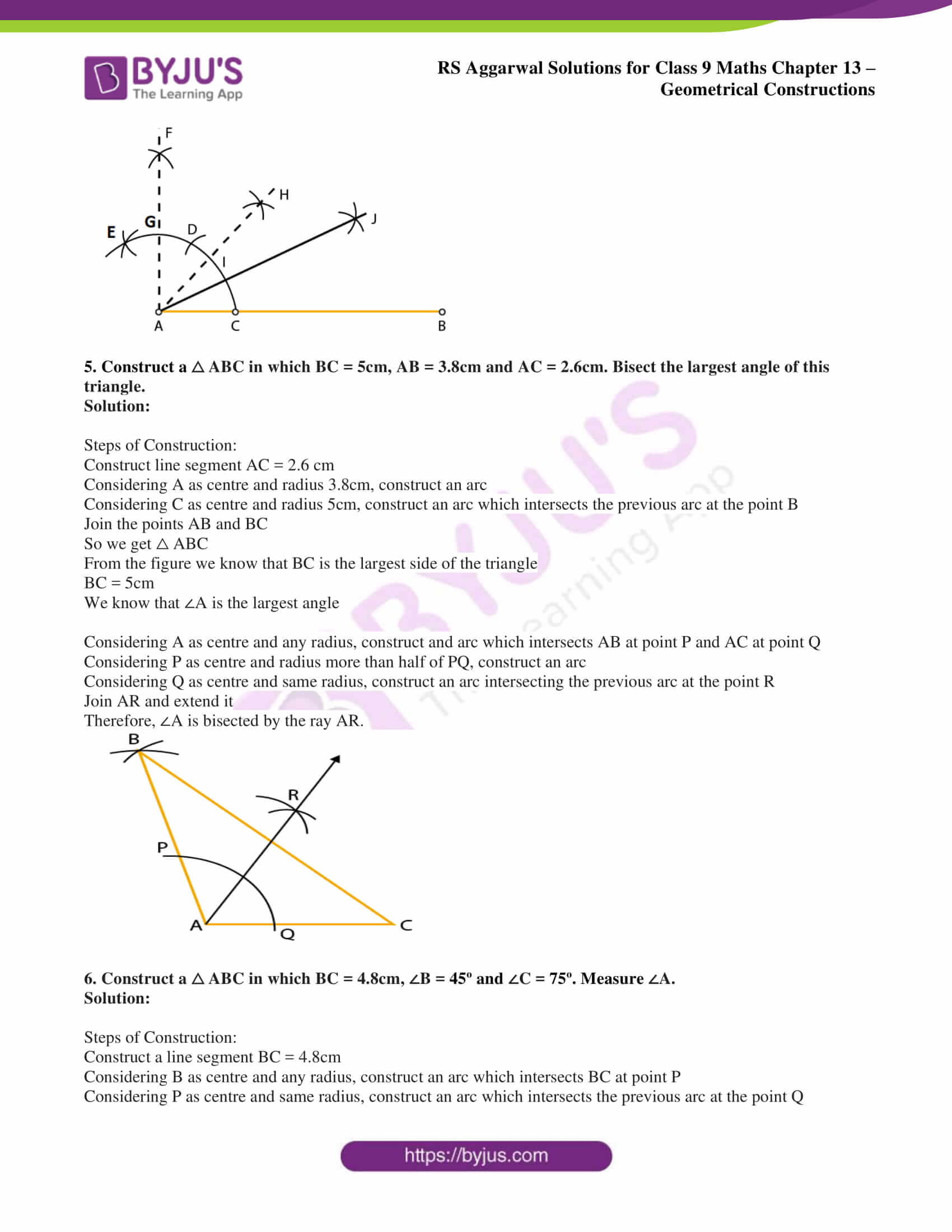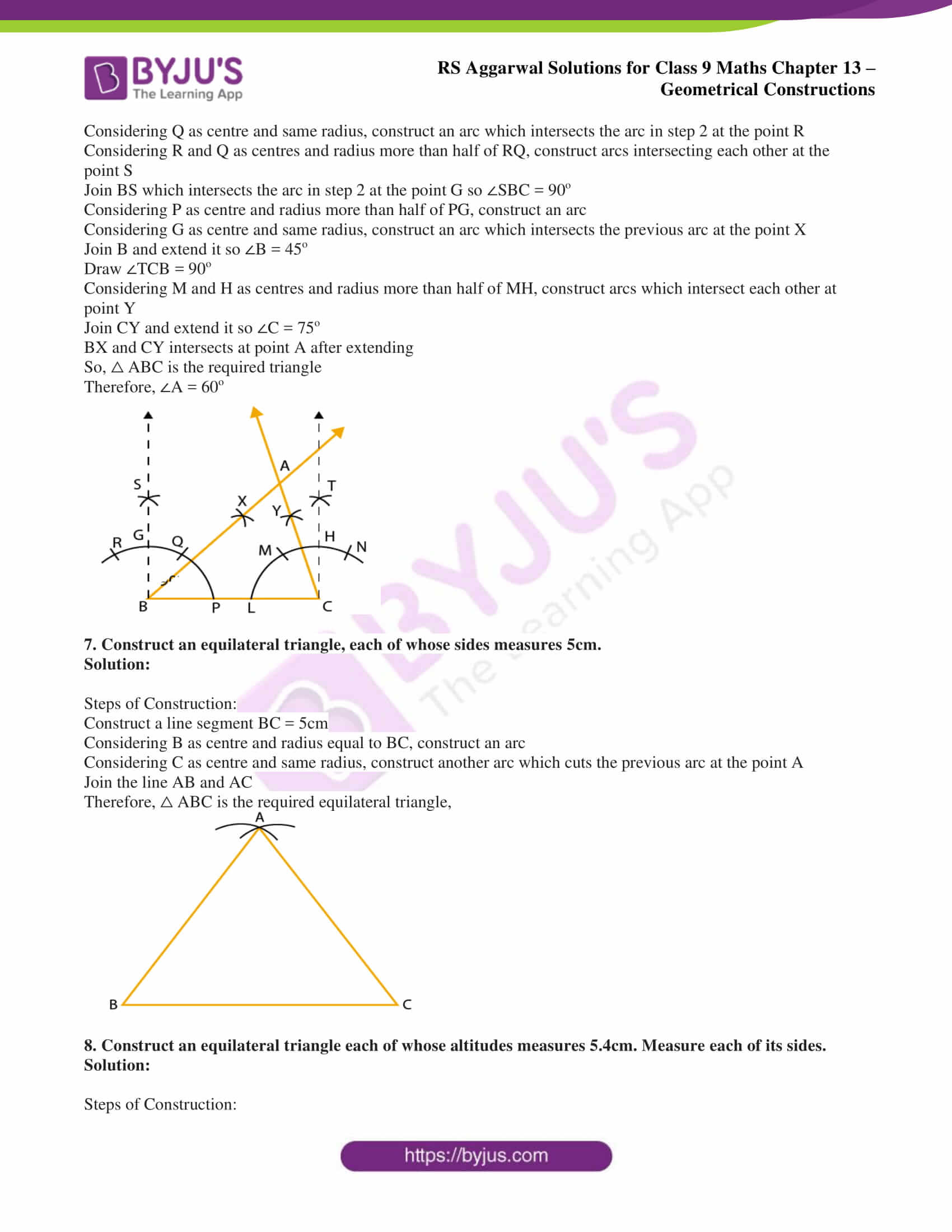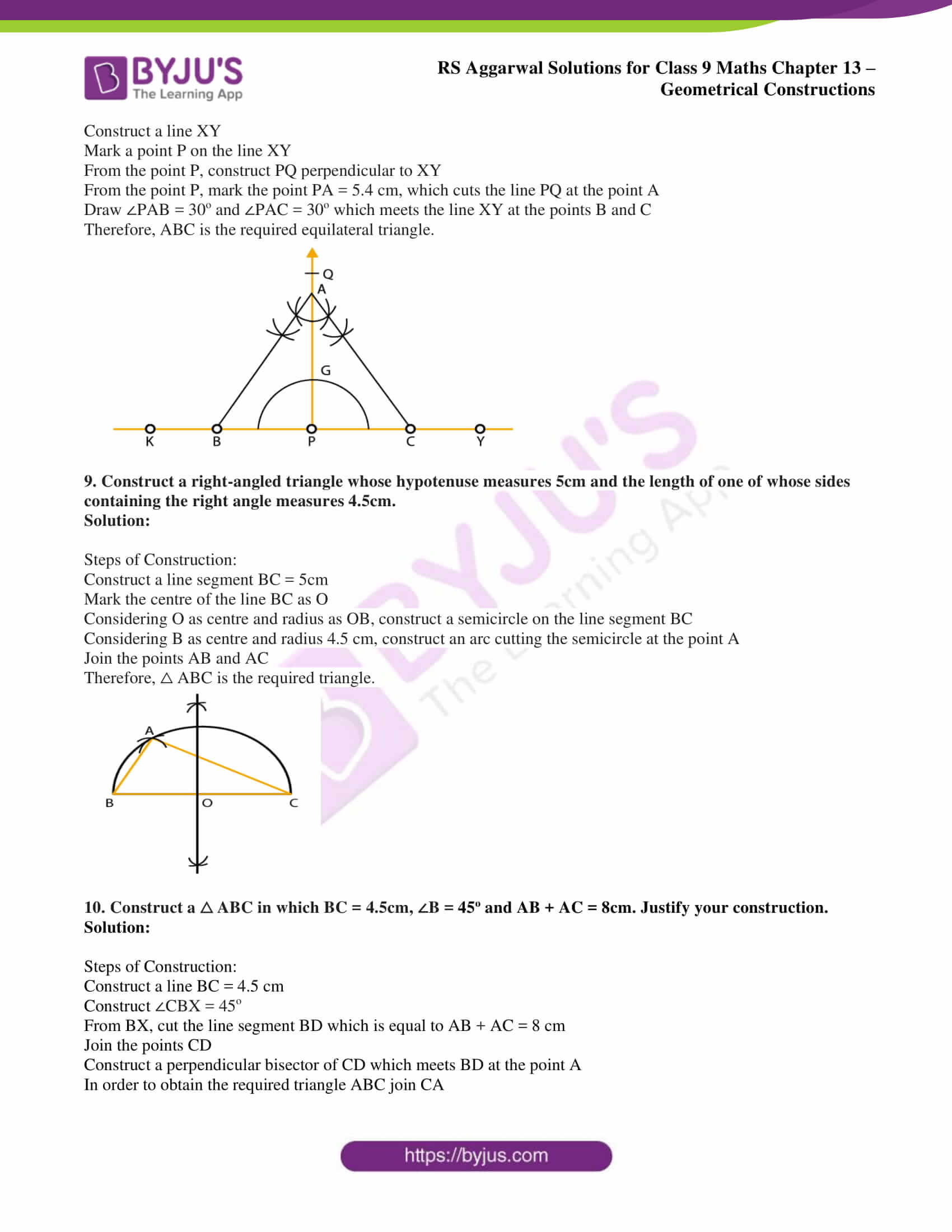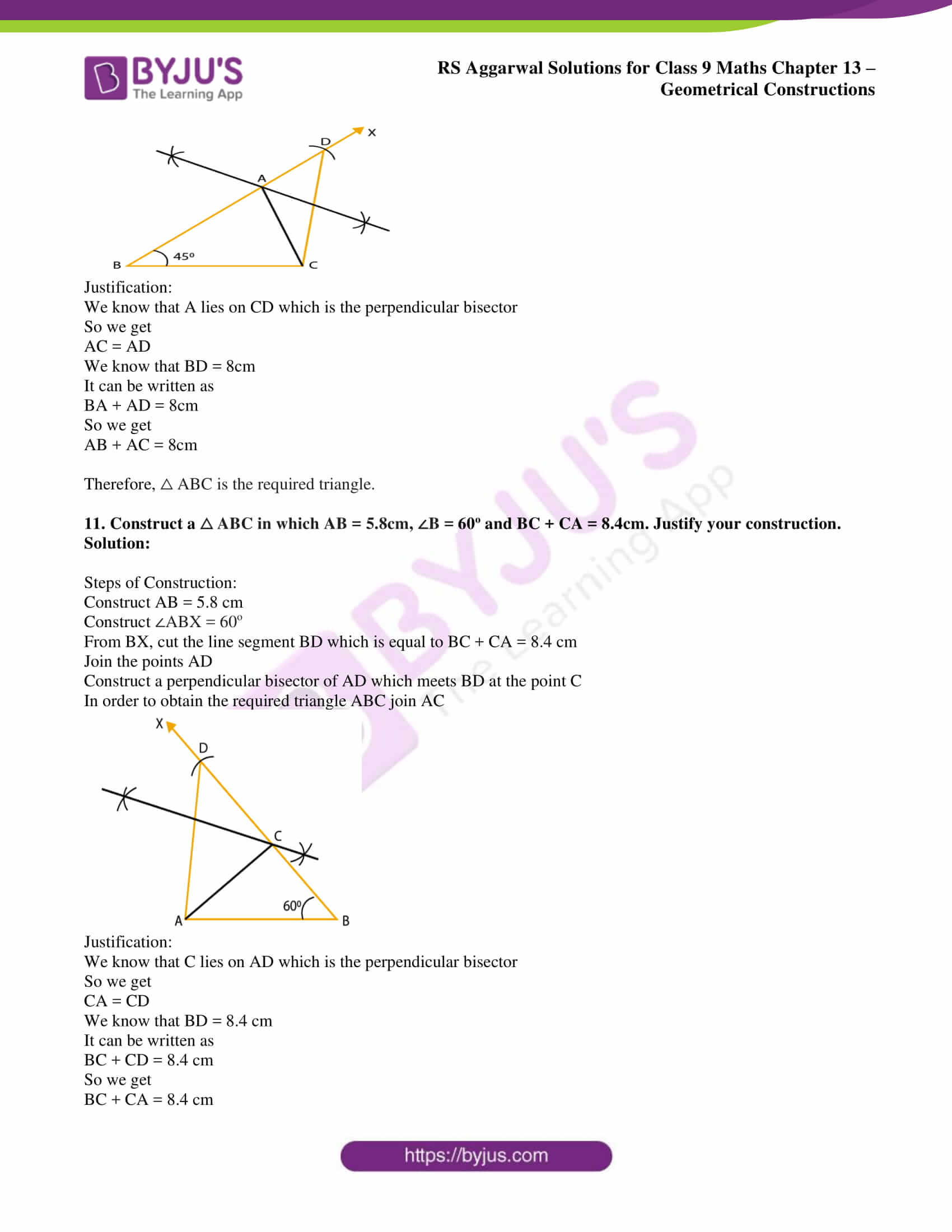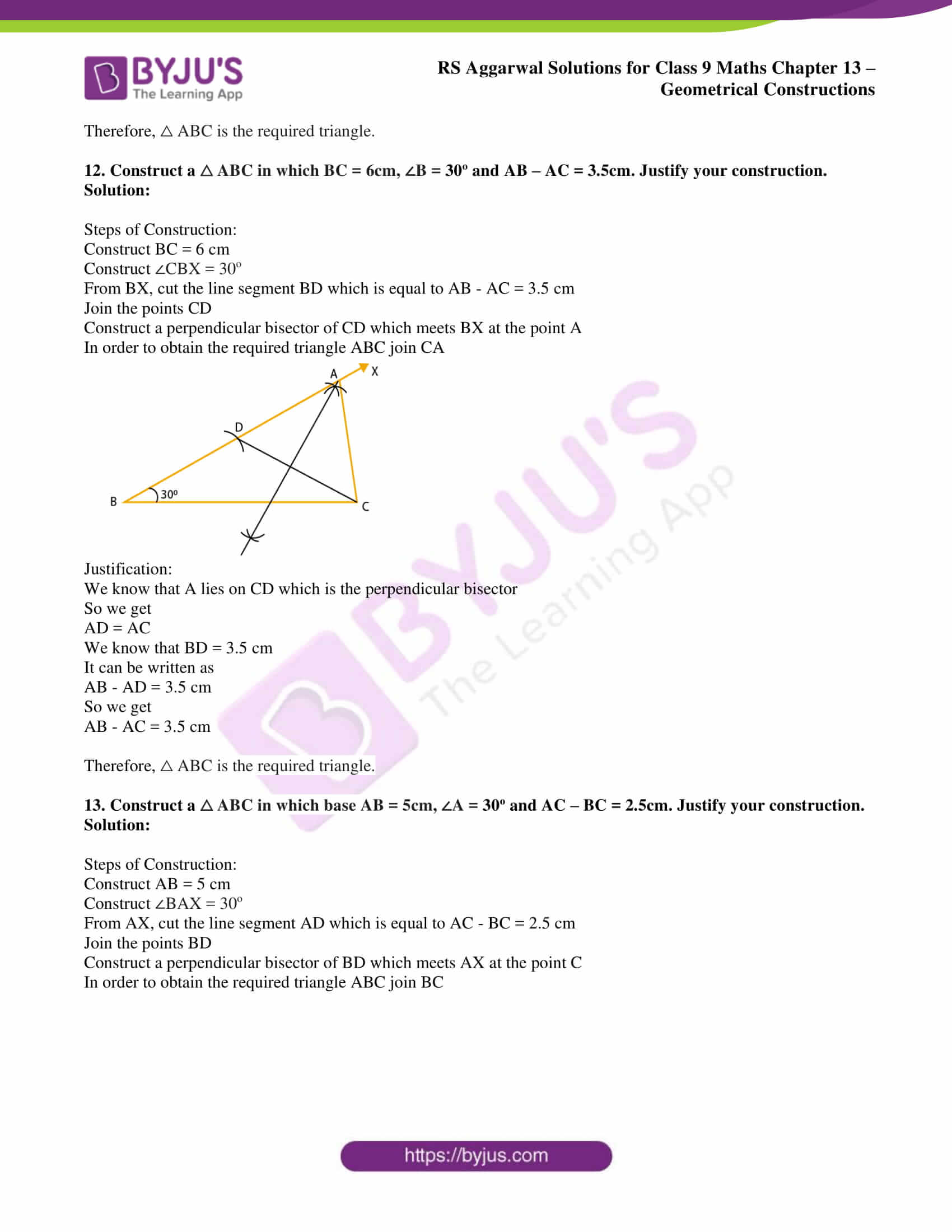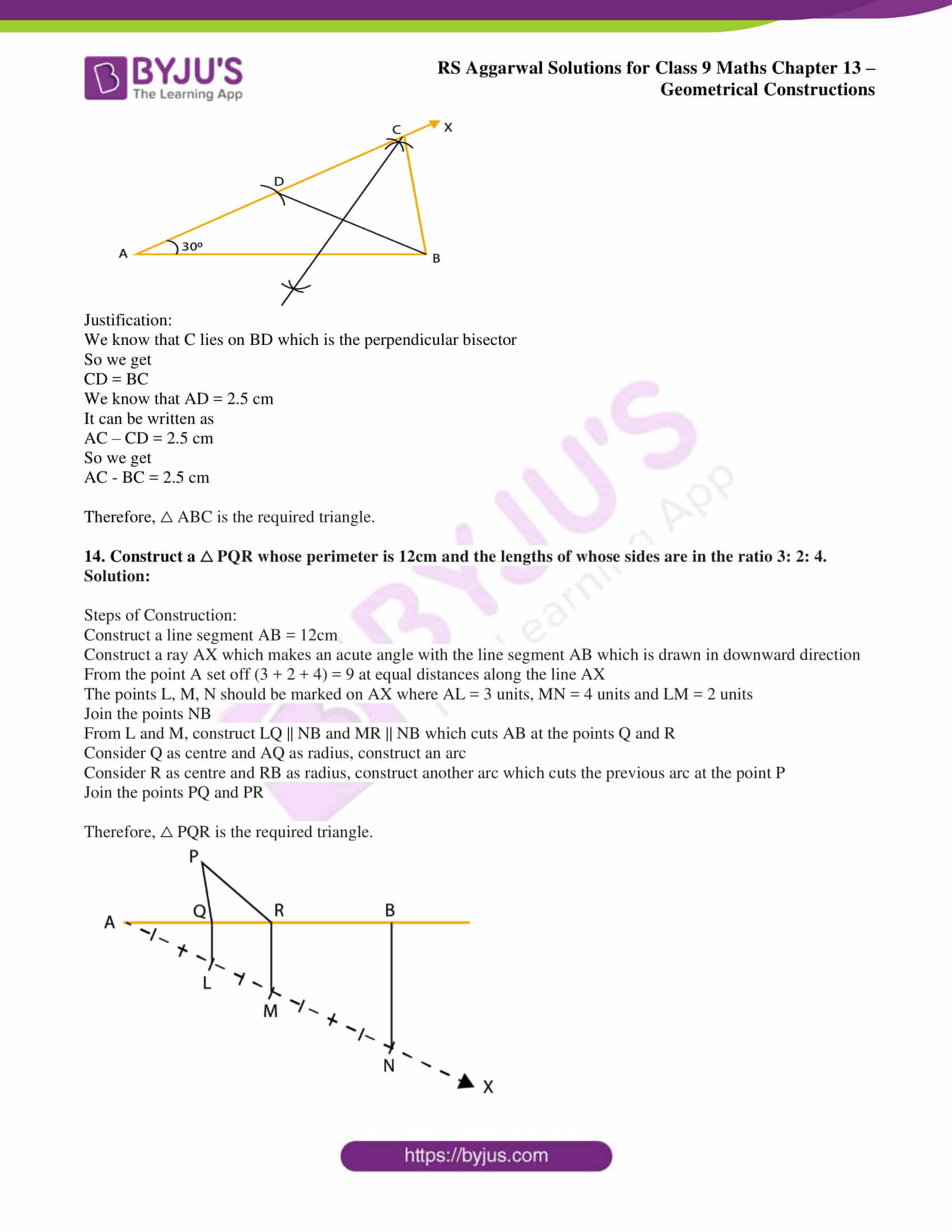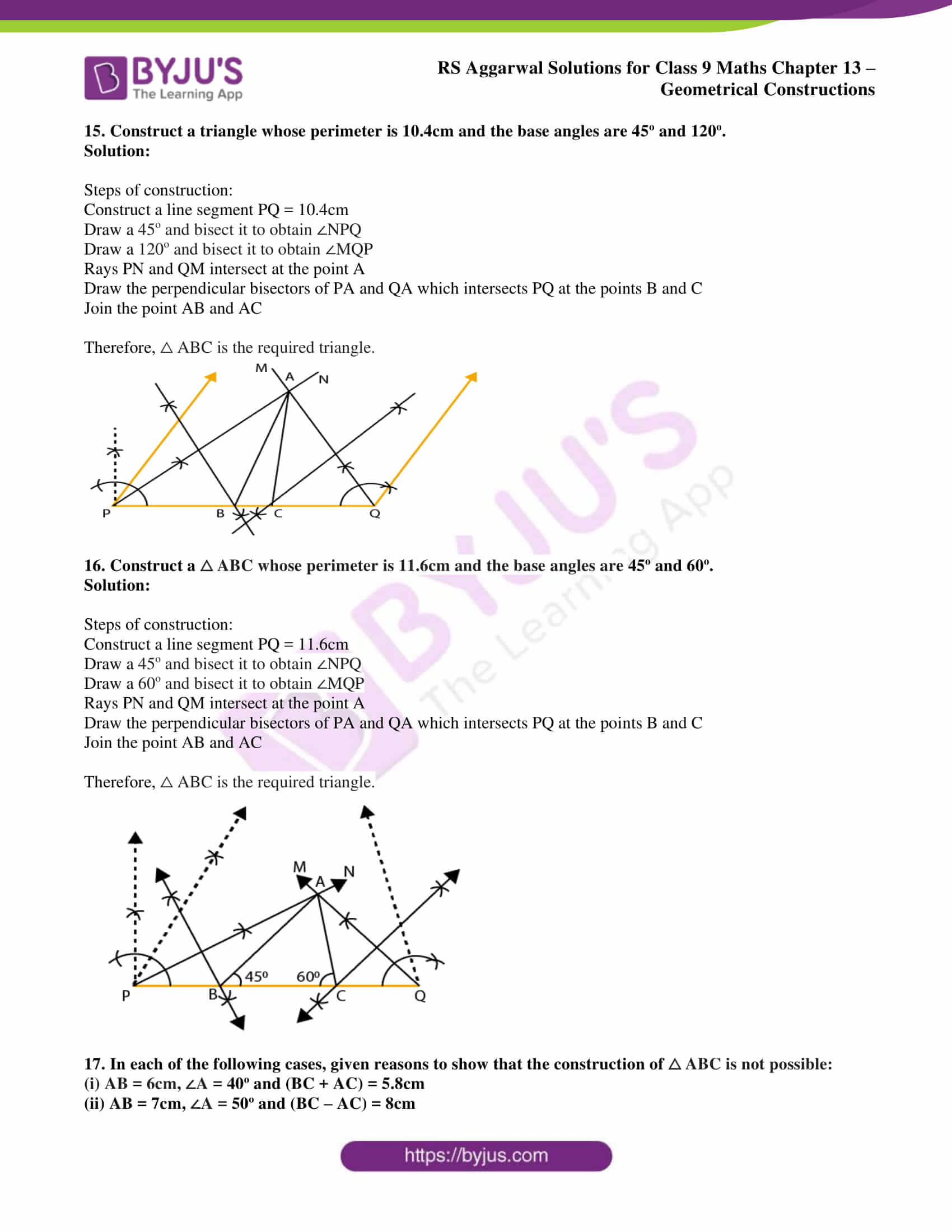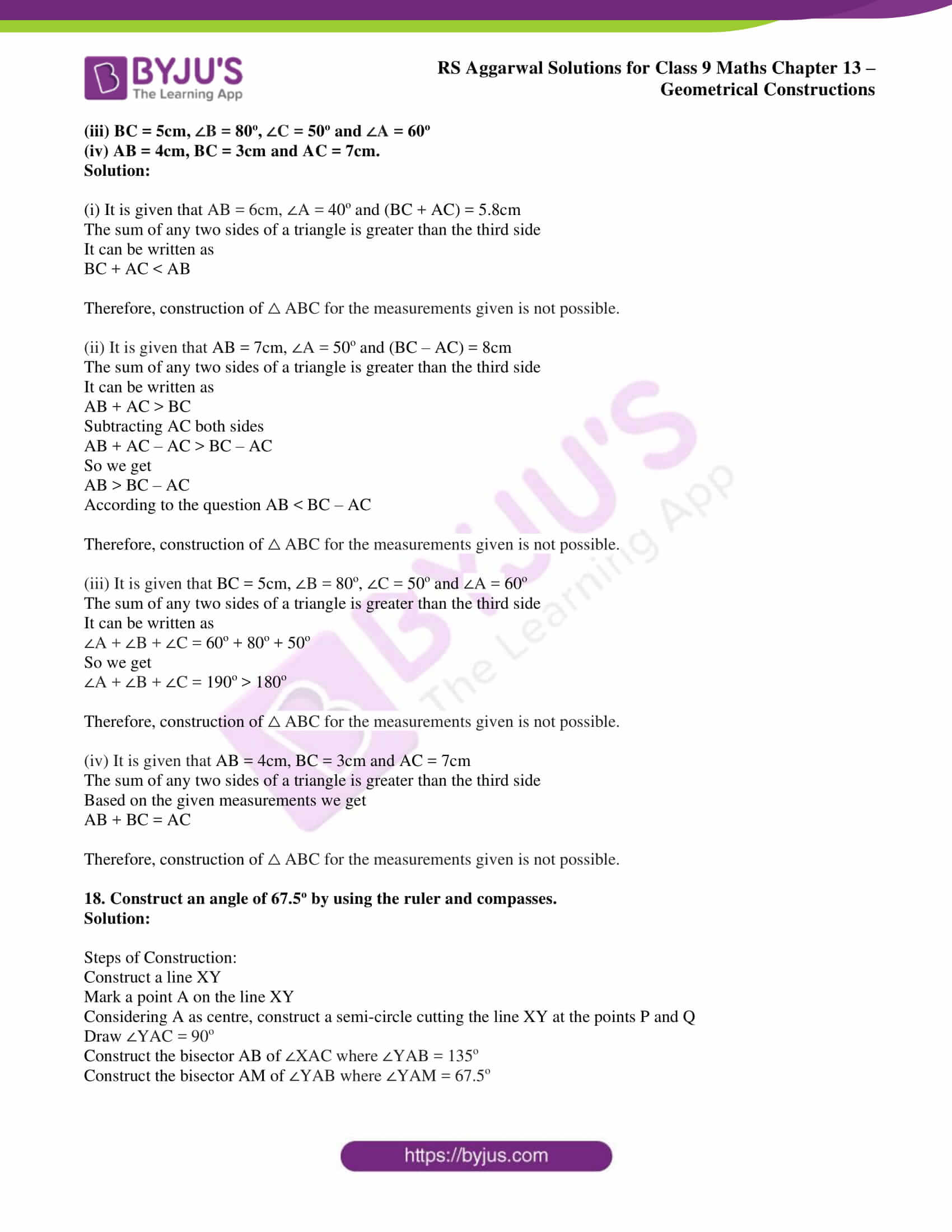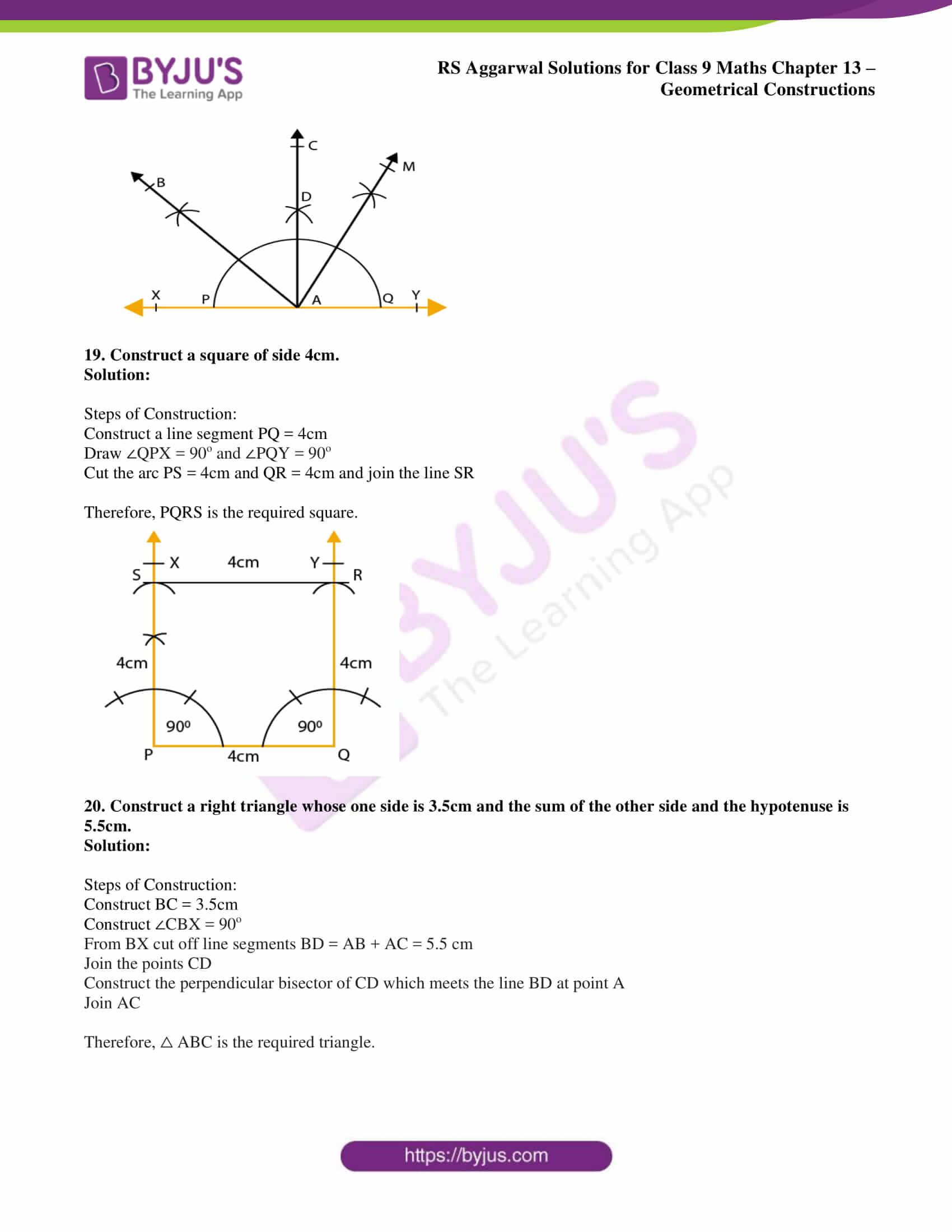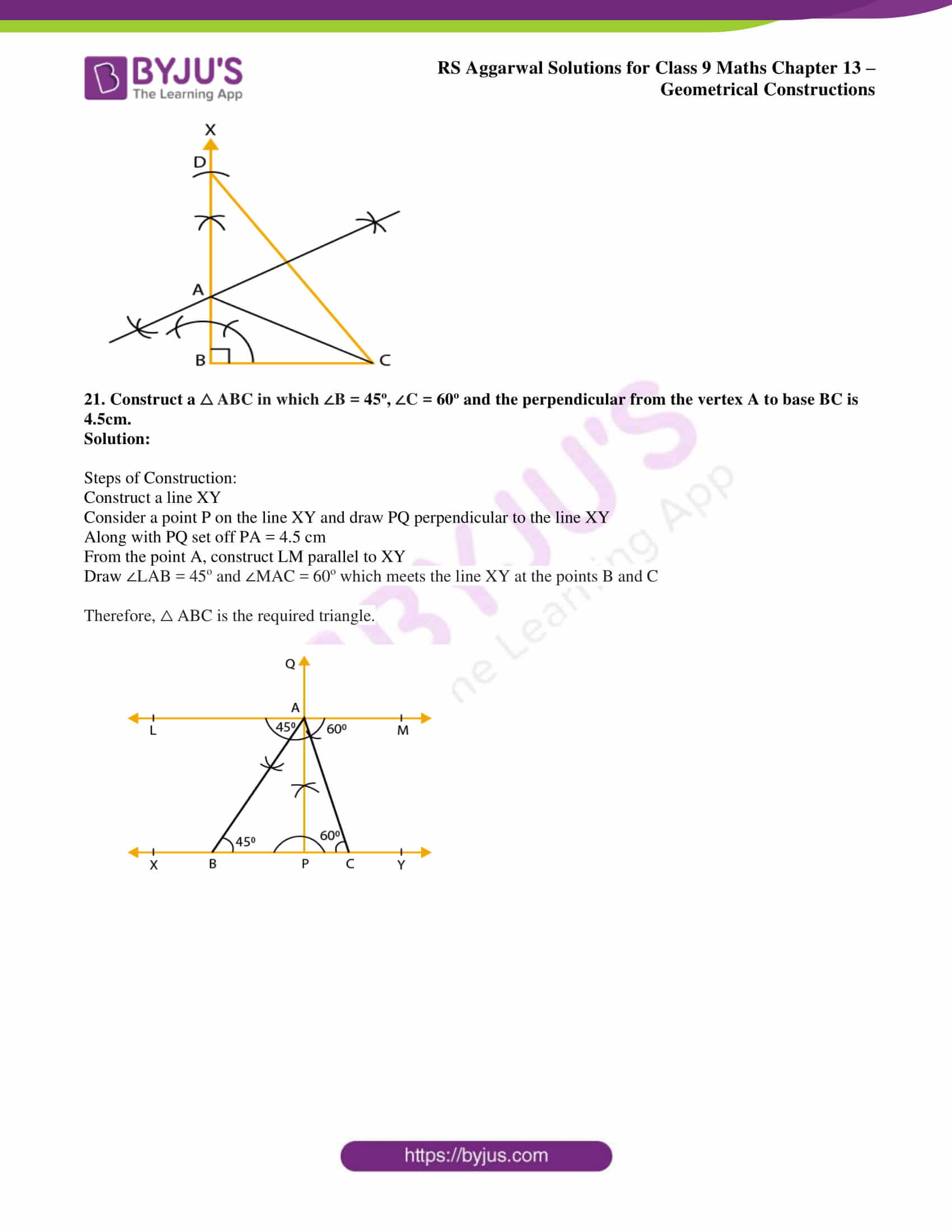## Access RS Aggarwal Solutions for Class 9 Maths Chapter 13: Geometrical Constructions

Exercise 13 PAGE: 514

1. Draw a line segment AB = 5.6 cm and draw its perpendicular bisector. Measure the length of each part.

Solution:

Steps of Construction:

Construct a line segment AB = 5.6 cm

Considering A as centre and radius more than half of line segment AB, draw two arcs on the each side of the line segment AB

Considering B as centre and radius more than half of line segment AB, draw arcs which cuts the previous arcs at the points P and Q

Join the points PQ such that it intersects the line segment AB at the point M

Therefore, PQ is the perpendicular bisector of the line segment AB.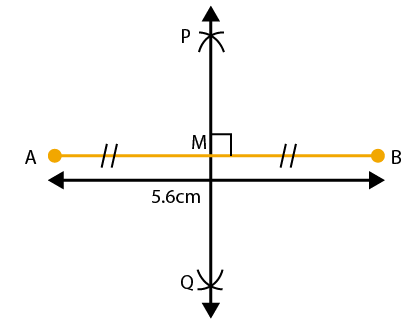So the length of each part is AM = BM = 2.8 cm

2. Draw an angle of 80o with the help of a protractor and bisect it. Measure each part of the bisected angle.

Solution:

Steps of Construction:

Construct a ray OB

Using the protractor construct ∠AOB of 80o

Considering O as centre and convenient radius construct an arc cutting the sides OA and OB at the points Q and P

Considering Q as centre and radius more than half of the angle PQ, construct an arc

Considering P as the centre and radius more than half of the angle PQ, construct another arc which intersects the previous arc at the point R

Join the points OR and produce it in order to form a ray OX

Therefore, OX is the required bisector of the ∠AOB

So the measure of each part of the bisected angle is ∠AOX = ∠BOX = 40o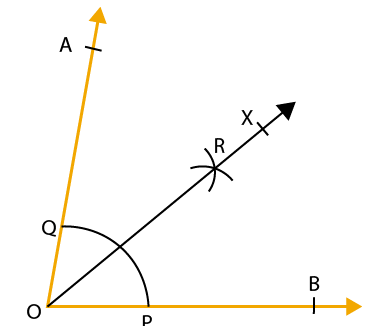3. Construct an angle of 90o using ruler and compasses and bisect it.

Solution:

Steps of Construction:

Construct a line segment OA

Considering O as centre and suitable radius construct an arc cutting the line OA at the point B

Considering B as centre and suitable radius as before construct an arc to cut the previous arc at the point C

Considering C as centre and the same radius cut the arc at point D

Considering C as centre and the radius more than half of CD construct an arc

Considering D as centre and same radius construct another arc to cut the previous arc at the point E

Join E so we get ∠AOE = 90o

Considering B as centre and radius more than half of CB construct an arc

Considering C as centre and same radius construct an arc which cuts the previous arc at the point F

Join OF

Therefore, F is the bisector of right ∠AOE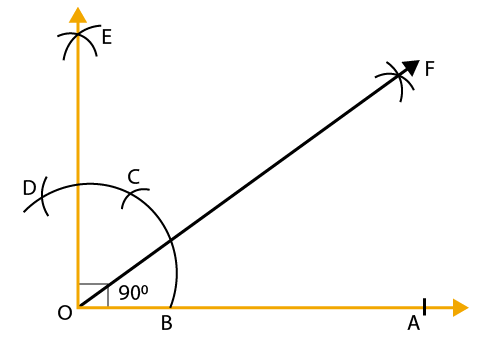4. Construct each of the following angles, using ruler and compasses:

(i) 75o

(ii) 37.5o

(iii) 135o

(iv) 105o

(v) 22.5o

Solution:

(i) Steps of Construction:

Construct a line segment PQ

Considering P as centre and any radius construct an arc which intersects the line PQ at the point R

Considering R as centre and same radius construct an arc which intersects the pervious arc at the point S

Considering S as centre and same radius construct an arc which intersects the pervious arc in step 2 at the point T

Considering T and S as centre and radius more than half of TS, construct arcs which intersects each other at the point U

Join the line PU which intersects the arc in step 2 at the point V

Considering V and S as centres and radius more than half of VS, construct arcs which intersects each other at the point W

Join the line PW

Therefore, we know that ∠WPQ = 75o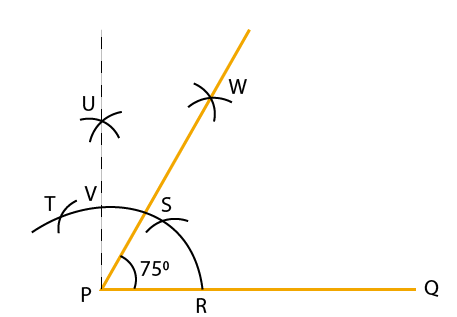(ii) Steps of Construction:

Construct a line segment PQ

Considering P as centre and any radius, construct an arc which intersects the line PQ at the point R

Considering R as centre and any radius, construct an arc which intersects the previous arc at the point S

Considering S as centre and same radius, construct an arc which intersects the arc in step 2 at the point T

Considering T and S as centre and radius more than half of TS, construct arcs which intersects each other at the point U

Join the points PU which intersects the arc in step 2 at the point V

Considering V and S as cenntres and radius more than half of VS, construct arcs which intersect each other at the point W

Join the points PW and ∠WPQ = 75o

Bisect the ∠WPQ

Therefore, we know that ∠ZPQ = 37.5o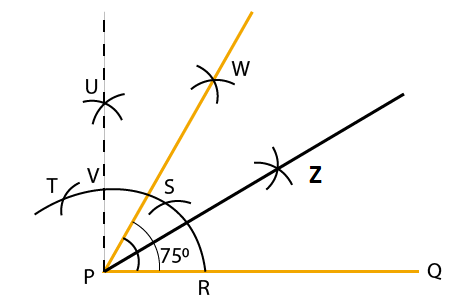(iii) Steps of construction:

Construct a line segment AB and produce BA to C

Considering A as centre and any radius, construct an arc intersecting AC at point D and AB at point E

Considering D and E as centres and radius more than half of DE, construct two arch intersecting each other at the point F

Join the points FA which intersects the arc in step 2 at point G

Considering G and D as centres and radius more than half of GD, construct two arcs intersecting each other at the point H

Join HA

Therefore, we know that ∠HAB = 135o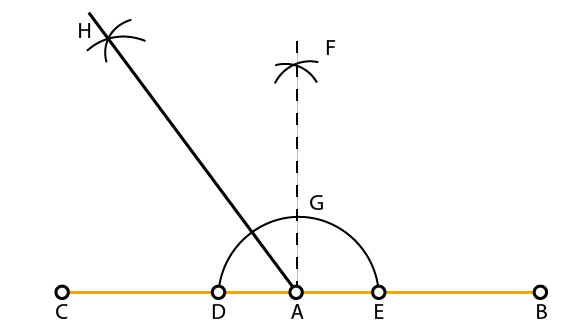(iv) Steps of Construction:

Construct a line segment PQ

Considering P as centre and any radius, construct an arc which intersects PQ at the point R

Considering R as centre and same radius, construct an arc which intersects the previous arc at the point S

Considering S as centre and same radius, construct an arc which intersects the arc in step 2 at the point T

Considering T and S as centres and radius more than half of TS, construct arcs which intersects each other at point U

Join PU which intersects the arc in step 2 at point V

Considering T and V as centres and radius more than half of TV, construct arcs intersecting each other at the point W

Join PW which is makes 105o with the ray PQ

Therefore, we know that ∠WPQ = 105o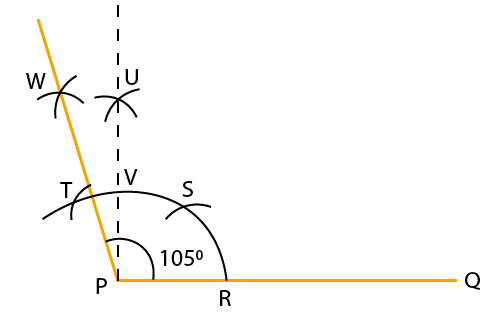(v) Steps of Construction:

Construct a line segment AB

Considering A as centre and any radius, construct an arc which intersects AB at the point C

Considering C as centre and same radius, construct an arc which intersects the previous arc at the point D

Considering D as centre and same radius, construct an arc which intersects the arc in step 2 at the point E

Considering E and D as centres and radius more than half of ED, construct arcs which intersect each other at the point F

Join the points AF which intersects the arc in step 2 at the point G

Considering G and C as centres and radius more than half of GC, construct arcs intersecting each other at the point H

Join AH which intersects the arc in step 2 at the point I

Considering I and C as centres and radius more than half of IC, construct arcs which intersect each other at the point J

Join AJ

Therefore, we know that ∠JAB = 22.5o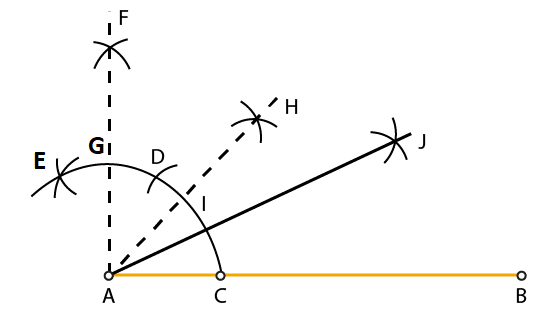5. Construct a △ ABC in which BC = 5cm, AB = 3.8cm and AC = 2.6cm. Bisect the largest angle of this triangle.

Solution:

Steps of Construction:

Construct line segment AC = 2.6 cm

Considering A as centre and radius 3.8cm, construct an arc

Considering C as centre and radius 5cm, construct an arc which intersects the previous arc at the point B

Join the points AB and BC

So we get △ ABC

From the figure we know that BC is the largest side of the triangle

BC = 5cm

We know that ∠A is the largest angle

Considering A as centre and any radius, construct and arc which intersects AB at point P and AC at point Q

Considering P as centre and radius more than half of PQ, construct an arc

Considering Q as centre and same radius, construct an arc intersecting the previous arc at the point R

Join AR and extend it

Therefore, ∠A is bisected by the ray AR.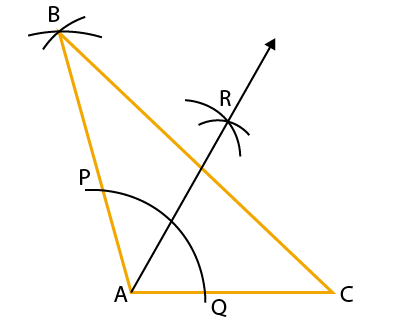6. Construct a △ ABC in which BC = 4.8cm, ∠B = 45o and ∠C = 75o. Measure ∠A.

Solution:

Steps of Construction:

Construct a line segment BC = 4.8cm

Considering B as centre and any radius, construct an arc which intersects BC at point P

Considering P as centre and same radius, construct an arc which intersects the previous arc at the point Q

Considering Q as centre and same radius, construct an arc which intersects the arc in step 2 at the point R

Considering R and Q as centres and radius more than half of RQ, construct arcs intersecting each other at the point S

Join BS which intersects the arc in step 2 at the point G so ∠SBC = 90o

Considering P as centre and radius more than half of PG, construct an arc

Considering G as centre and same radius, construct an arc which intersects the previous arc at the point X

Join B and extend it so ∠B = 45o

Draw ∠TCB = 90o

Considering M and H as centres and radius more than half of MH, construct arcs which intersect each other at point Y

Join CY and extend it so ∠C = 75o

BX and CY intersects at point A after extending

So, △ ABC is the required triangle

Therefore, ∠A = 60o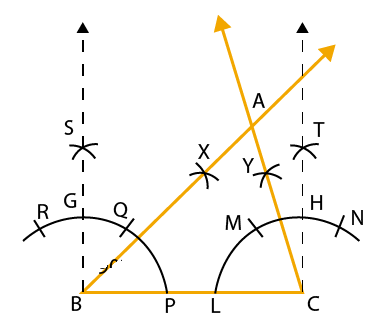7. Construct an equilateral triangle, each of whose sides measures 5cm.

Solution:

Steps of Construction:

Construct a line segment BC = 5cm

Considering B as centre and radius equal to BC, construct an arc

Considering C as centre and same radius, construct another arc which cuts the previous arc at the point A

Join the line AB and AC

Therefore, △ ABC is the required equilateral triangle,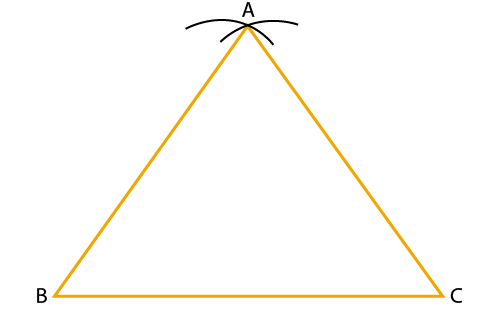8. Construct an equilateral triangle each of whose altitudes measures 5.4cm. Measure each of its sides.

Solution:

Steps of Construction:

Construct a line XY

Mark a point P on the line XY

From the point P, construct PQ perpendicular to XY

From the point P, mark the point PA = 5.4 cm, which cuts the line PQ at the point A

Draw ∠PAB = 30o and ∠PAC = 30o which meets the line XY at the points B and C

Therefore, ABC is the required equilateral triangle.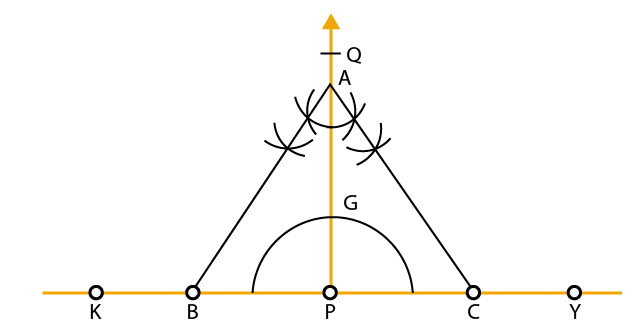9. Construct a right-angled triangle whose hypotenuse measures 5cm and the length of one of whose sides containing the right angle measures 4.5cm.

Solution:

Steps of Construction:

Construct a line segment BC = 5cm

Mark the centre of the line BC as O

Considering O as centre and radius as OB, construct a semicircle on the line segment BC

Considering B as centre and radius 4.5 cm, construct an arc cutting the semicircle at the point A

Join the points AB and AC

Therefore, △ ABC is the required triangle.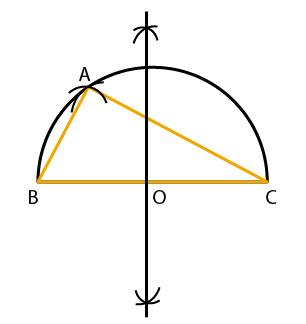10. Construct a △ ABC in which BC = 4.5cm, ∠B = 45o and AB + AC = 8cm. Justify your construction.

Solution:

Steps of Construction:

Construct a line BC = 4.5 cm

Construct ∠CBX = 45o

From BX, cut the line segment BD which is equal to AB + AC = 8 cm

Join the points CD

Construct a perpendicular bisector of CD which meets BD at the point A

In order to obtain the required triangle ABC join CA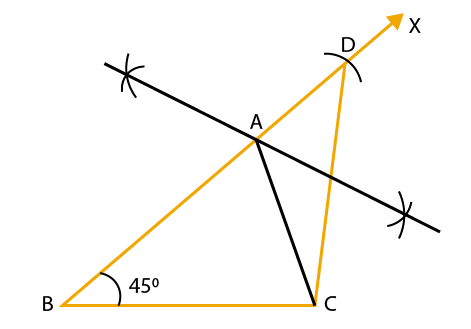Justification:

We know that A lies on CD which is the perpendicular bisector

So we get

We know that BD = 8cm

It can be written as

So we get

AB + AC = 8cm

Therefore, △ ABC is the required triangle.

11. Construct a △ ABC in which AB = 5.8cm, ∠B = 60o and BC + CA = 8.4cm. Justify your construction.

Solution:

Steps of Construction:

Construct AB = 5.8 cm

Construct ∠ABX = 60o

From BX, cut the line segment BD which is equal to BC + CA = 8.4 cm

Construct a perpendicular bisector of AD which meets BD at the point C

In order to obtain the required triangle ABC join AC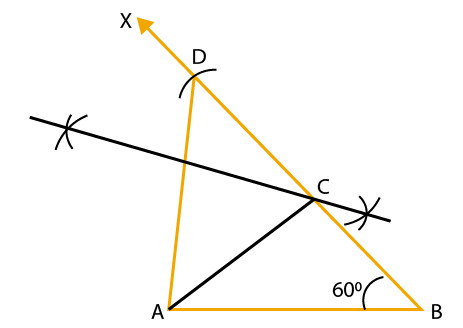Justification:

We know that C lies on AD which is the perpendicular bisector

So we get

CA = CD

We know that BD = 8.4 cm

It can be written as

BC + CD = 8.4 cm

So we get

BC + CA = 8.4 cm

Therefore, △ ABC is the required triangle.

12. Construct a △ ABC in which BC = 6cm, ∠B = 30o and AB – AC = 3.5cm. Justify your construction.

Solution:

Steps of Construction:

Construct BC = 6 cm

Construct ∠CBX = 30o

From BX, cut the line segment BD which is equal to AB – AC = 3.5 cm

Join the points CD

Construct a perpendicular bisector of CD which meets BX at the point A

In order to obtain the required triangle ABC join CA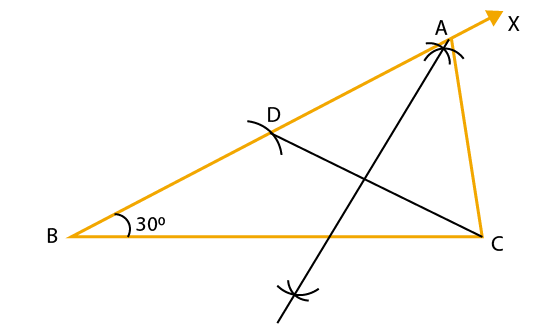Justification:

We know that A lies on CD which is the perpendicular bisector

So we get

We know that BD = 3.5 cm

It can be written as

AB – AD = 3.5 cm

So we get

AB – AC = 3.5 cm

Therefore, △ ABC is the required triangle.

13. Construct a △ ABC in which base AB = 5cm, ∠A = 30o and AC – BC = 2.5cm. Justify your construction.

Solution:

Steps of Construction:

Construct AB = 5 cm

Construct ∠BAX = 30o

From AX, cut the line segment AD which is equal to AC – BC = 2.5 cm

Join the points BD

Construct a perpendicular bisector of BD which meets AX at the point C

In order to obtain the required triangle ABC join BC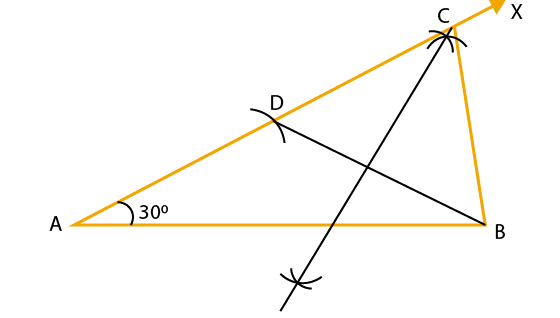Justification:

We know that C lies on BD which is the perpendicular bisector

So we get

CD = BC

We know that AD = 2.5 cm

It can be written as

AC – CD = 2.5 cm

So we get

AC – BC = 2.5 cm

Therefore, △ ABC is the required triangle.

14. Construct a △ PQR whose perimeter is 12cm and the lengths of whose sides are in the ratio 3: 2: 4.

Solution:

Steps of Construction:

Construct a line segment AB = 12cm

Construct a ray AX which makes an acute angle with the line segment AB which is drawn in downward direction

From the point A set off (3 + 2 + 4) = 9 at equal distances along the line AX

The points L, M, N should be marked on AX where AL = 3 units, MN = 4 units and LM = 2 units

Join the points NB

From L and M, construct LQ || NB and MR || NB which cuts AB at the points Q and R

Consider Q as centre and AQ as radius, construct an arc

Consider R as centre and RB as radius, construct another arc which cuts the previous arc at the point P

Join the points PQ and PR

Therefore, △ PQR is the required triangle.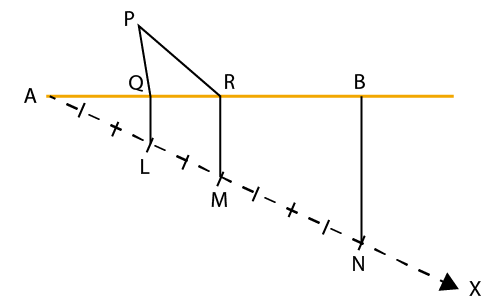15. Construct a triangle whose perimeter is 10.4cm and the base angles are 45o and 120o.

Solution:

Steps of construction:

Construct a line segment PQ = 10.4cm

Draw a 45o and bisect it to obtain ∠NPQ

Draw a 120o and bisect it to obtain ∠MQP

Rays PN and QM intersect at the point A

Draw the perpendicular bisectors of PA and QA which intersects PQ at the points B and C

Join the point AB and AC

Therefore, △ ABC is the required triangle.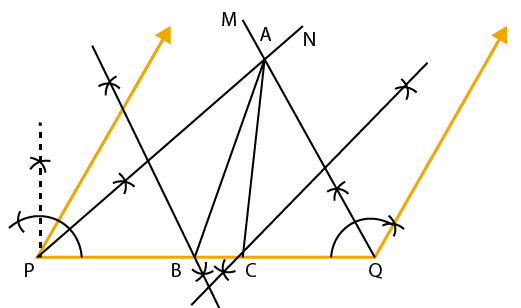16. Construct a △ ABC whose perimeter is 11.6cm and the base angles are 45o and 60o.

Solution:

Steps of construction:

Construct a line segment PQ = 11.6cm

Draw a 45o and bisect it to obtain ∠NPQ

Draw a 60o and bisect it to obtain ∠MQP

Rays PN and QM intersect at the point A

Draw the perpendicular bisectors of PA and QA which intersects PQ at the points B and C

Join the point AB and AC

Therefore, △ ABC is the required triangle.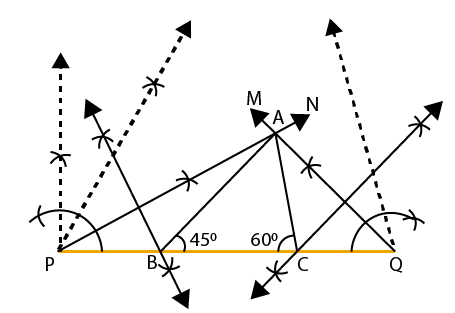17. In each of the following cases, given reasons to show that the construction of △ ABC is not possible:

(i) AB = 6cm, ∠A = 40o and (BC + AC) = 5.8cm

(ii) AB = 7cm, ∠A = 50o and (BC – AC) = 8cm

(iii) BC = 5cm, ∠B = 80o, ∠C = 50o and ∠A = 60o

(iv) AB = 4cm, BC = 3cm and AC = 7cm.

Solution:

(i) It is given that AB = 6cm, ∠A = 40o and (BC + AC) = 5.8cm

The sum of any two sides of a triangle is greater than the third side

It can be written as

BC + AC < AB

Therefore, construction of △ ABC for the measurements given is not possible.

(ii) It is given that AB = 7cm, ∠A = 50o and (BC – AC) = 8cm

The sum of any two sides of a triangle is greater than the third side

It can be written as

AB + AC > BC

Subtracting AC both sides

AB + AC – AC > BC – AC

So we get

AB > BC – AC

According to the question AB < BC – AC

Therefore, construction of △ ABC for the measurements given is not possible.

(iii) It is given that BC = 5cm, ∠B = 80o, ∠C = 50o and ∠A = 60o

The sum of any two sides of a triangle is greater than the third side

It can be written as

∠A + ∠B + ∠C = 60o + 80o + 50o

So we get

∠A + ∠B + ∠C = 190o > 180o

Therefore, construction of △ ABC for the measurements given is not possible.

(iv) It is given that AB = 4cm, BC = 3cm and AC = 7cm

The sum of any two sides of a triangle is greater than the third side

Based on the given measurements we get

AB + BC = AC

Therefore, construction of △ ABC for the measurements given is not possible.

18. Construct an angle of 67.5o by using the ruler and compasses.

Solution:

Steps of Construction:

Construct a line XY

Mark a point A on the line XY

Considering A as centre, construct a semi-circle cutting the line XY at the points P and Q

Draw ∠YAC = 90o

Construct the bisector AB of ∠XAC where ∠YAB = 135o

Construct the bisector AM of ∠YAB where ∠YAM = 67.5o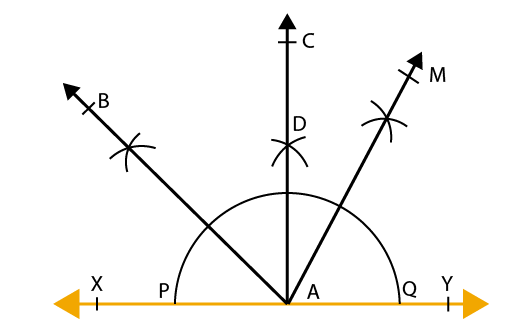19. Construct a square of side 4cm.

Solution:

Steps of Construction:

Construct a line segment PQ = 4cm

Draw ∠QPX = 90o and ∠PQY = 90o

Cut the arc PS = 4cm and QR = 4cm and join the line SR

Therefore, PQRS is the required square.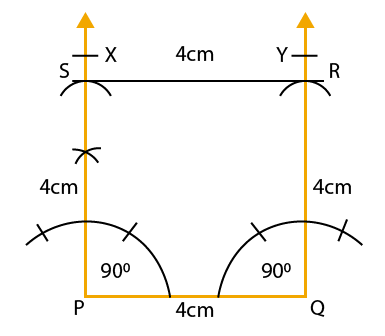20. Construct a right triangle whose one side is 3.5cm and the sum of the other side and the hypotenuse is 5.5cm.

Solution:

Steps of Construction:

Construct BC = 3.5cm

Construct ∠CBX = 90o

From BX cut off line segments BD = AB + AC = 5.5 cm

Join the points CD

Construct the perpendicular bisector of CD which meets the line BD at point A

Join AC

Therefore, △ ABC is the required triangle.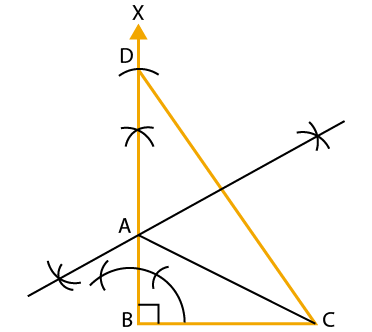21. Construct a △ ABC in which ∠B = 45o, ∠C = 60o and the perpendicular from the vertex A to base BC is 4.5cm.

Solution:

Steps of Construction:

Construct a line XY

Consider a point P on the line XY and draw PQ perpendicular to the line XY

Along with PQ set off PA = 4.5 cm

From the point A, construct LM parallel to XY

Draw ∠LAB = 45o and ∠MAC = 60o which meets the line XY at the points B and C

Therefore, △ ABC is the required triangle.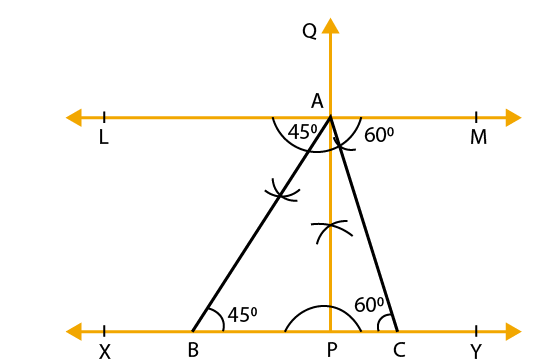### RS Aggarwal Solutions for Class 9 Maths Chapter 13: Geometrical Constructions

Chapter 13, Geometrical Constructions, has 1 exercise which contains problems based on line segments. The important concepts based on the RS Aggarwal Solutions Chapter 13 are as given below:

• Perpendicular bisector of a line segment
• To draw the perpendicular bisector of a given line segment
• Bisector of a given angle
• Construction of angles using ruler and compasses

### RS Aggarwal Solutions Class 9 Maths Chapter 13 – Geometrical Constructions

PDF format of the solutions can be downloaded by the students and used as a vital resource to score well in the exams. RS Aggarwal Solutions is based on the textbook of current CBSE syllabus and evaluation process. The problems are solved in an elaborate manner as each step carries marks in the exam.

Geometrical Constructions are used by the architects to design a building with base, height and blueprint. It also has applications like mapping, navigation, surveying, calculate distances and astronomy. The students can access the PDF and refer them to solve the RS Aggarwal textbook based on the CBSE guidelines.